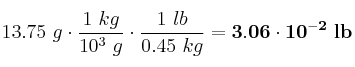# Mass of a volume of ethanol, expressed by pounds

, por

The density of ethanol is 0.79 g/mL. Calculate the mass, expressed in pounds, of 17.4 mL of ethanol, knowing that one pound is equal to 0.45 kg. Express the result using scientific notation.

## SOLUCIÓN:

We are going to solve this exercise in two steps. The first step will be to use the definition of density in order to calculate the mass of ethanol. The second step will be to do a change of units.
The mass of ethanol is:Now, we have to use two conversion factors: